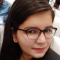## Recursive Programs to find Minimum and Maximum elements of array in C++Sunidhi Bansal

Updated on 03-Nov-2021 05:07:30

We are given an integer array Arr[] as input. The goal is to find maximum and minimum elements among the array using recursive methods.Since we are using recursion, we will traverse the whole array till we reach length=1 then return A which forms the base case. Else compare current element ... Read More

## Recursive Program for Binary to Decimal in C++Sunidhi Bansal

Updated on 03-Nov-2021 05:03:49

We are given a string containing a binary number. The goal is to find the equivalent decimal number using the recursive method.A binary number can be converted to decimal using following method-: Traverse from LSB to MSB and multiply each with power of 2i Where 0

## Recursive program to print formula for GCD of n integers in C++Sunidhi Bansal

Updated on 02-Nov-2021 08:29:54

We are given an integer as input. The goal is to print the formula of GCD of n numbers using recursion.We know that the GCD of three numbers say a1, b1 and c1 will be gcd(a1, gcd(b1, c1)).Similarly for more than three numbers, gcd can be obtained by formula as ... Read More

## Recursive program to check if number is palindrome or not in C++Sunidhi Bansal

Updated on 02-Nov-2021 08:28:12

We are given an integer as input. The goal is to find whether the input number Num is a palindrome or not using recursion.To check if a number is palindrome, reverse that number and check if both numbers are the same or not. If the reversed number is equal to ... Read More

## Recursive program for prime number in C++Sunidhi Bansal

Updated on 02-Nov-2021 08:26:06

We are given an integer as input. The goal is to find whether the input number Num is a prime or non-prime using recursion.To check if a number is prime or not, start traversing from i=2 to i

## Recursive Implementation of atoi() in C++Sunidhi Bansal

Updated on 02-Nov-2021 08:20:05

We are given a string containing a number. The goal is to find the equivalent number using the recursive atoi() method. int atoi(const char *str) converts the string argument str to an integer (type int).Example-:Input − Str[] = "58325"Output − The Equivalent decimal is :58325Explanation − The string contains the equivalent number 58325Input − ... Read More

## Recursive function to do substring search in C++Sunidhi Bansal

Updated on 02-Nov-2021 08:18:19

Given two strings Str and subStr as input. The goal is to find whether text present in subStr exists in Str as substring or not. The string X is called a substring of Y if whole X is present in Y at least once. We will use a recursive approach ... Read More

## C++ Program Recursive Insertion SortSunidhi Bansal

Updated on 02-Nov-2021 08:14:39

Insertion Sort is one of the sorting algorithms used to sort data by inserting elements like a deck of cards. All the elements are arranged from left to right then considering the first one as already sorted, insert rest to the sorted list on the left. Each element is compared ... Read More

## Recursive function to check if a string is palindrome in C++Sunidhi Bansal

Updated on 02-Nov-2021 08:09:17

We are given a string Str as input. The goal is to find if the input string is a palindrome word or not using a recursive function. Palindrome strings are those strings that when read from front or end form the same word. The strings of length 0 are considered ... Read More

## C Program for Recursive Bubble SortSunidhi Bansal

Updated on 02-Nov-2021 07:58:22

Bubble Sort is one of the simplest sorting algorithms used to sort data by comparing the adjacent elements. All the elements are compared in phases. The first phase places the largest value at the end, the second phase places the second largest element at the second last position and so ... Read More

Previous 1 ... 3 4 5 6 7 ... 111 Next